## Recap

In the previous chapter:

• We defined stochastic games;
• We investigated approached to obtaining Markov strategy Nash equilibria.

In this chapter we will take a look at a very different type of game.

## Matching Games

Consider the following situation:

“In a population of $$N$$ suitors and $$N$$ reviewers. We allow the suitors and reviewers to rank their preferences and are now trying to match the suitors and reviewers in such a way as that every matching is stable.”

If we consider the following example with suitors: $$S=\{a,b,c\}$$ and reviewers: $$R=\{A,B,C\}$$ with preferences shown.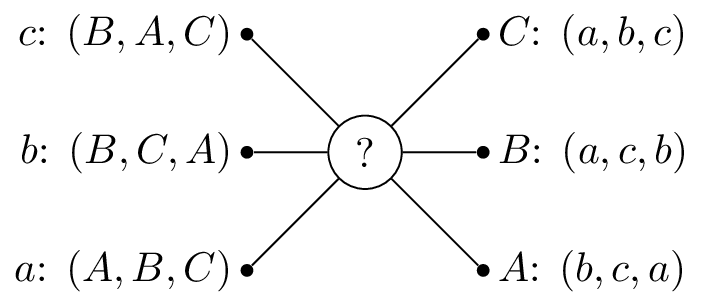So that $$A$$ would prefer to be matched with $$b$$, then $$c$$ and lastly $$c$$. One possible matching would be is shown.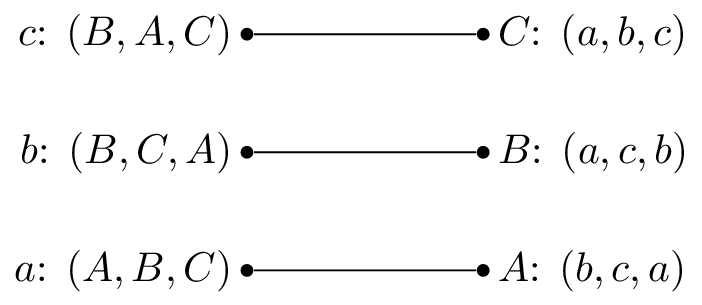In this situation, $$a$$ and $$b$$ are getting their first choice and $$c$$ their second choice. However $$B$$ actually prefers $$c$$ so that matching is unstable.

Let us write down some formal definitions:

### Definition of a matching game

A matching game of size $$N$$ is defined by two disjoint sets $$S$$ and $$R$$ or suitors and reviewers of size $$N$$. Associated to each element of $$S$$ and $$R$$ is a preference list:

A matching $$M$$ is a any bijection between $$S$$ and $$R$$. If $$s\in S$$ and $$r\in R$$ are matched by $$M$$ we denote:

### Definition of a blocking pair

A pair $$(s,r)$$ is said to block a matching $$M$$ if $$M(s)\ne r$$ but $$s$$ prefers $$r$$ to $$M(s)$$ and $$r$$ prefers $$s$$ to $$M^{-1}(r)$$.

In our previous example $$(c,B)$$ blocks the proposed matching.

### Definition of a stable matching

A matching $$M$$ with no blocking pair is said to be stable.

A stable matching is shown.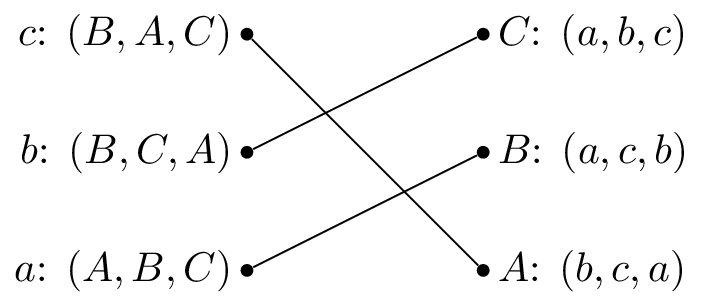The stable matching is not unique, the matching shown is also stable: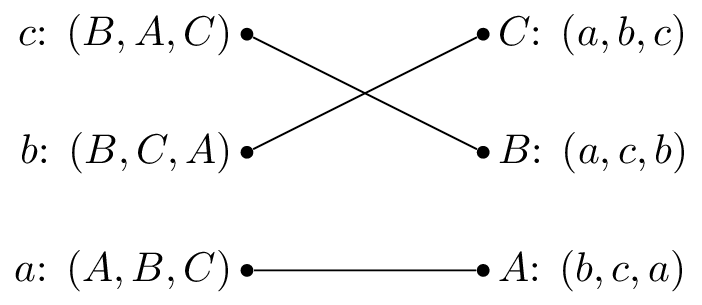## The Gale-Shapley Algorithm

Here is the Gale-Shapley algorithm, which gives a stable matching for a matching game:

1. Assign every $$s\in S$$ and $$r\in R$$ to be unmatched
2. Pick some unmatched $$s\in S$$, let $$r$$ be the top of $$s$$’s preference list:
• If $$r$$ is unmatched set $$M(s)=r$$
• If $$r$$ is matched:
• If $$r$$ prefers $$s$$ to $$M^{-1}(r)$$ then set $$M(r)=s$$
• Otherwise $$s$$ remains unmatched and remove $$r$$ from $$s$$’s preference list.
3. Repeat step 2 until all $$s\in S$$ are matched.

Let us illustrate this algorithm with the above example.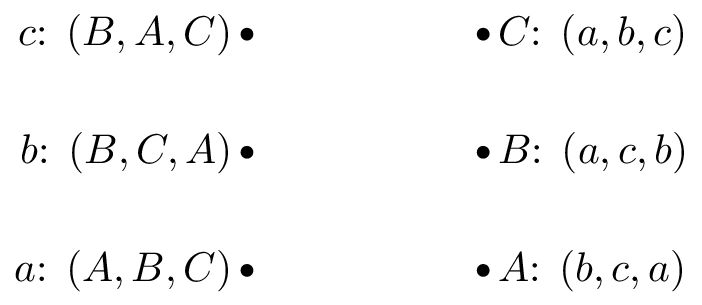We pick $$c$$ and as all the reviewers are unmatched set $$M(c)=B$$.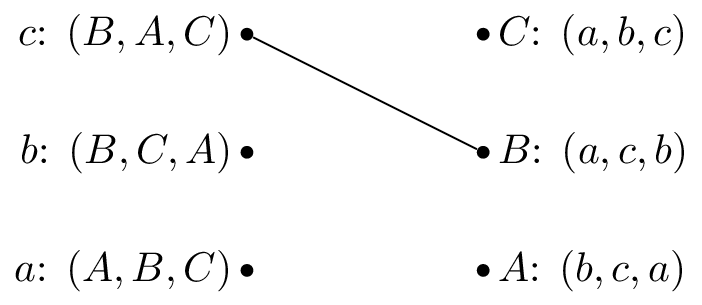We pick $$b$$ and as $$B$$ is matched but prefers $$c$$ to $$b$$ we cross out $$B$$ from $$b$$’s preferences.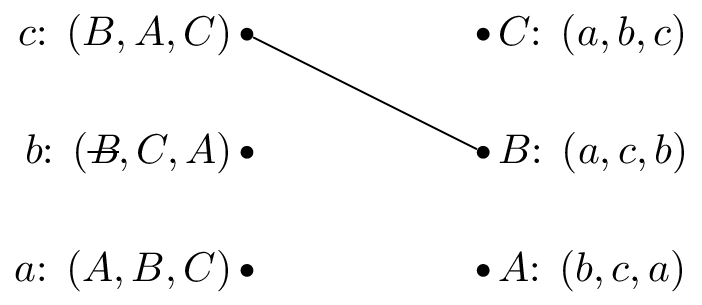We pick $$b$$ again and set $$M(b)=C$$.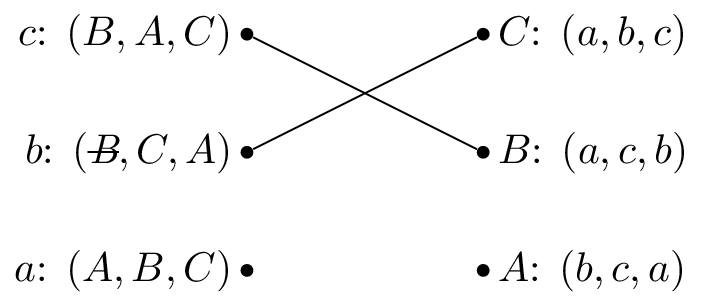We pick $$a$$ and set $$M(a)=A$$.Let us repeat the algorithm but pick $$b$$ as our first suitor.We pick $$b$$ and as all the reviewers are unmatched set $$M(b)=B$$.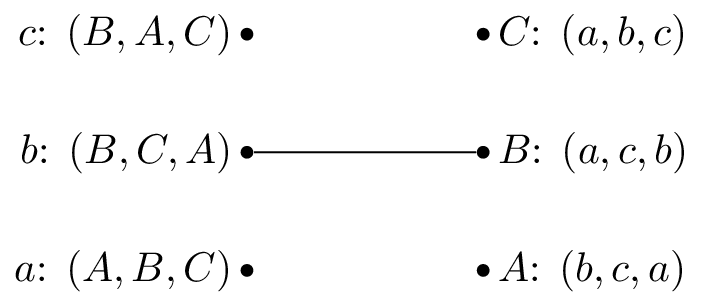We pick $$a$$ and as $$A$$ is unmatched set $$M(a)=A$$.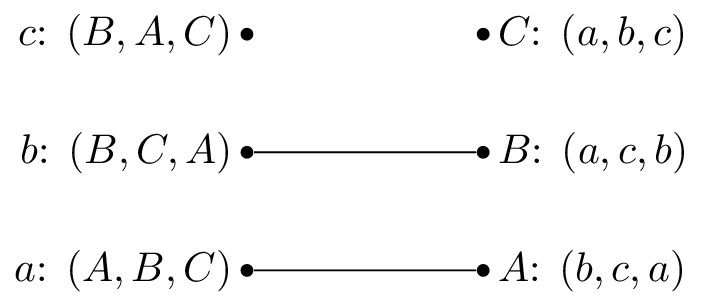We pick $$c$$ and $$b$$ is matched but prefers $$c$$ to $$M^{-1}(B)=b$$, we set $$M(c)=B$$.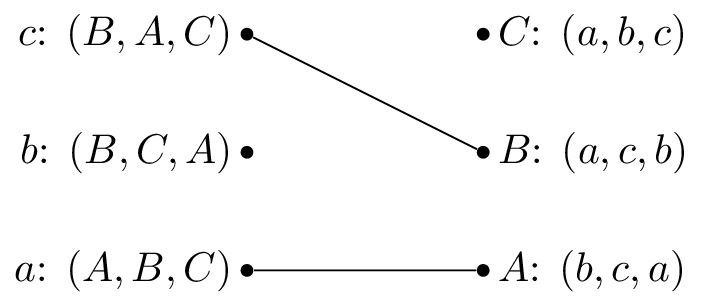We pick $$b$$ and as $$B$$ is matched but prefers $$c$$ to $$b$$ we cross out $$B$$ from $$b$$’s preferences: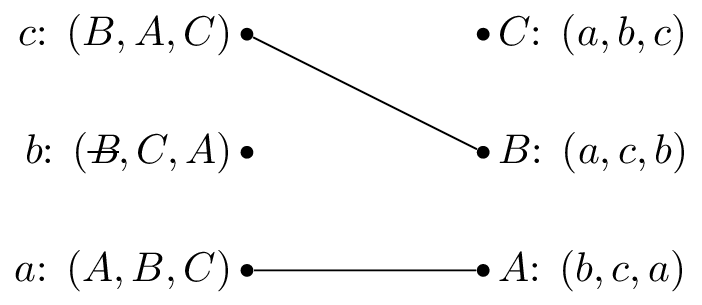We pick $$b$$ again and set $$M(b)=C$$.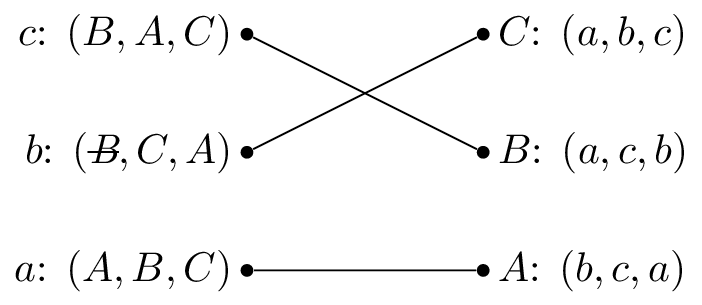Both these have given the same matching.

### Theorem guaranteeing a unique matching as output of the Gale Shapley algorithm.

All possible executions of the Gale-Shapley algorithm yield the same stable matching and in this stable matching every suitor has the best possible partner in any stable matching.

### Proof

Suppose that an arbitrary execution $$\alpha$$ of the algorithm gives $$M$$ and that another execution $$\beta$$ gives $$M’$$ such that $$\exists$$ $$s\in S$$ such that $$s$$ prefers $$r’=M’(s)$$ to $$r=M(s)$$.

Without loss of generality this implies that during $$\alpha$$ $$r’$$ must have rejected $$s$$. Suppose, again without loss of generality that this was the first occasion that a rejection occured during $$\alpha$$ and assume that this rejection occurred because $$r’=M(s’)$$. This implies that $$s’$$ has no stable match that is higher in $$s’$$’s preference list than $$r’$$ (as we have assumed that this is the first rejection).

Thus $$s’$$ prefers $$r’$$ to $$M’(s’)$$ so that $$(s’,r’)$$ blocks $$M’$$. Each suitor is therefore matched in $$M$$ with his favorite stable reviewer and since $$\alpha$$ was arbitrary it follows that all possible executions give the same matching.

We call a matching obtained from the Gale Shapley algorithm suitor-optimal because of the previous theorem. The next theorem shows another important property of the algorithm.

### Theorem of reviewer sub optimality

In a suitor-optimal stable matching each reviewer has the worst possible matching.

### Proof

Assume that the result is not true. Let $$M_0$$ be a suitor-optimal matching and assume that there is a stable matching $$M’$$ such that $$\exists$$ $$r$$ such that $$r$$ prefers $$s=M_0^{-1}(r)$$ to $$s’=M’^{-1}(r)$$. This implies that $$(r,s)$$ blocks $$M’$$ unless $$s$$ prefers $$M’(s)$$ to $$M_0(s)$$ which contradicts the fact the $$s$$ has no stable match that they prefer in $$M_0$$.